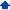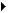### Creating matrices in equations

You can create column vectors, determinants, matrices, and other tabular layouts in equations. For example, you can create three-element row and column vectors, 2×2 or 3×3 matrices, or variable-sized matrices using the templates available in Equation Editor. The first nine templates allow you to create vectors and matrices with common sizes. The last three templates let you create a custom matrix or table using the following options.

##### Column align

This option positions entries horizontally in each column, according to the alignment you choose.

##### Equal column width

This option adjusts column widths so that each column is the same width as the widest column. If you do not enable this option, Equation Editor determines the width of each column individually.

##### Row align

This option positions entries vertically in each row, according to the alignment you choose.

##### Equal row height

This option adjusts the row heights so that each row is the same height as the tallest row. If you do not enable this option, Equation Editor determines the height of each row individually.

##### Row and column spacing

You can adjust row and column spacing. You can change the Matrix Column Spacing and Matrix Row Spacing parameters.

##### Partition lines, tables, and boxes

You can create various types of lines to partition the matrix by clicking the gaps between the matrix cells. Clicking a gap once produces a solid line. Clicking the gap again changes the solid line to a dashed line. Clicking the gap three times changes the dashed line to a dotted line, and clicking the gap four times removes the partition line. You can also create lines around the matrix by clicking around the outside of the matrix cells. By placing lines between matrix cells and around the outside of matrices, you can construct tables. You can also enclose an equation in a box by creating a 1×1 matrix with solid lines around its edges and creating an equation within the single matrix slot.

#### To insert a matrix in an equation1 Select an equation in the document.
 2 Click Edit(Open) equation object.
 3 Click the Edit equation tab.
 4 Click where you want to insert the matrix.
 5 Open the template picker at the bottom right, and click a matrix template.
 6 If the chosen template is a variable size matrix, fill in the appropriate matrix settings.
 7 Click OK.
 8 Click in each slot, and add the equation elements you want.